# AP物理1选择题真题讲解第二期

AP物理1选择题练习

1AP Physics Practice Exam 1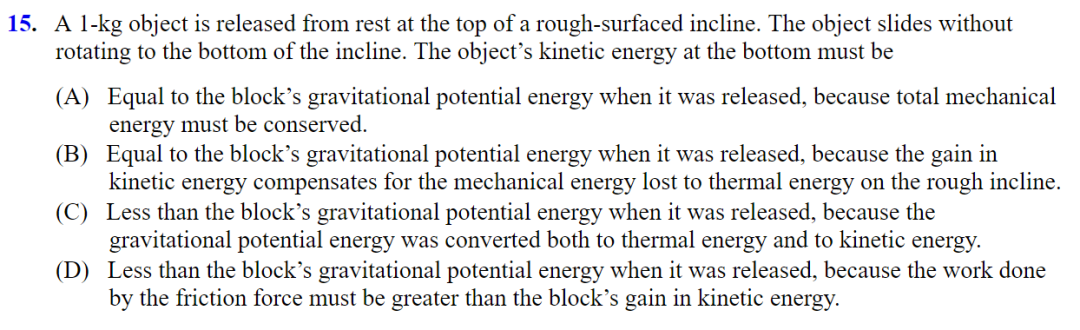C—Mechanical energy is not conserved because of the work done by the nonconservative friction force provided by the rough-surfaced incline. The object starts with only gravitational potential energy. This gravitational energy is converted to thermal energy via the friction force, and kinetic energy because the object speeds up.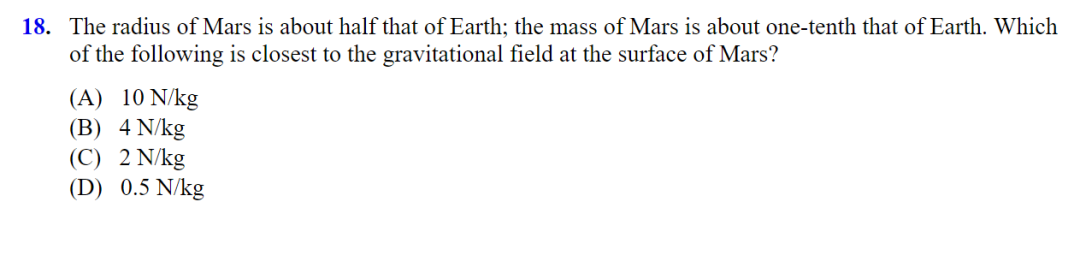B—The numerator for Mars is 1/10 that of Earth, reducing the gravitational field by 1/10. The denominator for Mars is (1/2)2 = 1/4 that of Earth. The overall gravitational field is multiplied by 4/10. On Earth, the gravitational field is 10 N/kg, so on Mars, g = 4 N/kg.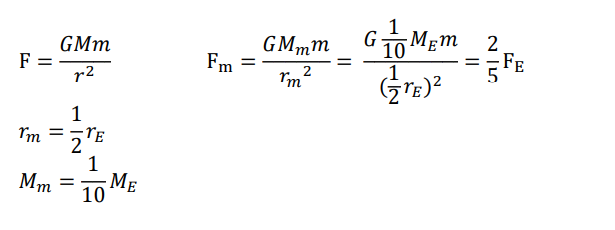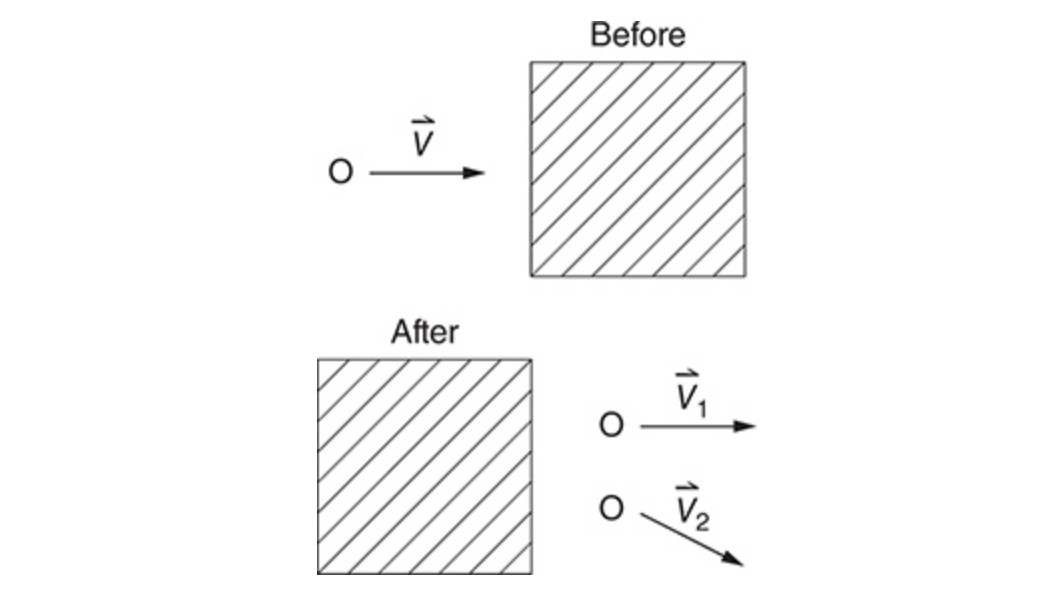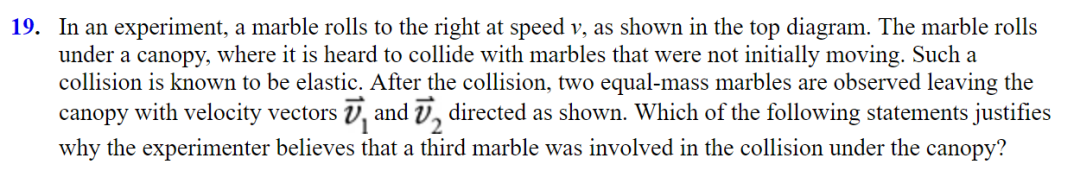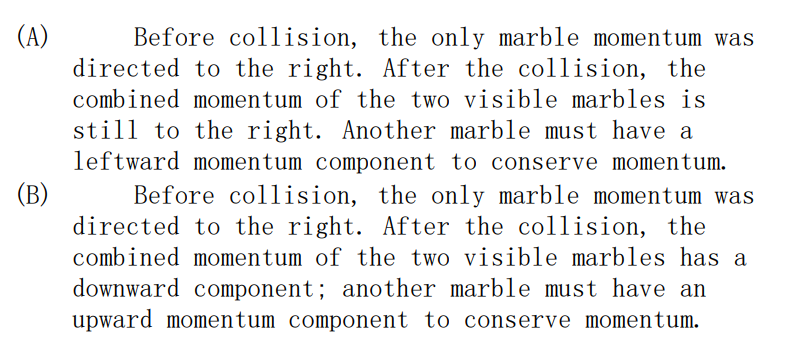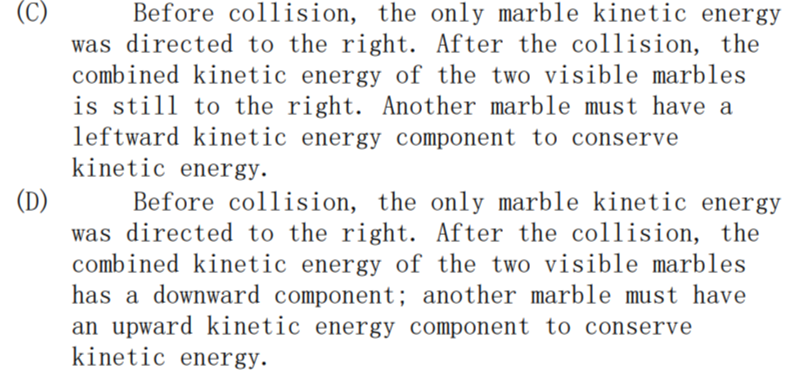B—Choices C and D are wrong because kinetic energy doesn’t have a direction. Choice A is wrong because momentum conservation does not require a leftward momentum component—since the initial momentum was all to the right, the final momentum should be to the right. It’s the vertical momentum that’s the problem. Since the vertical momentum was zero to start with, any vertical momentum after a collision must cancel out.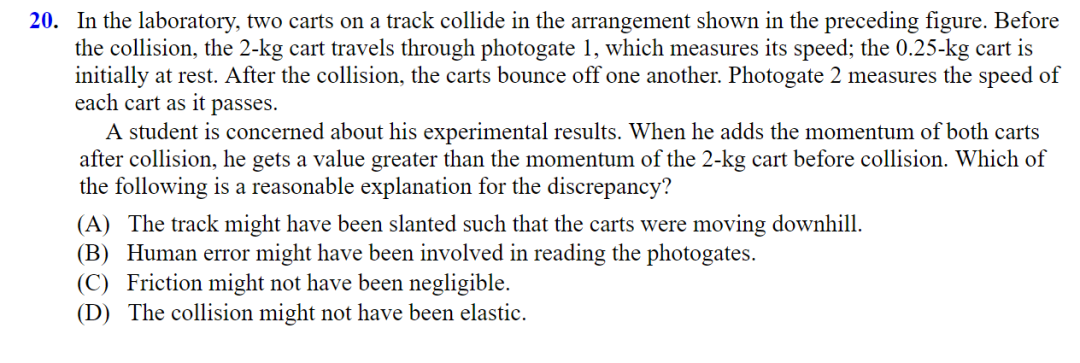A—for C, friction should reduce, not increase, the momentum. for D,The elasticity of a collision refers to kinetic energy conservation, not momentum conservation. For A, If the track is slanted downhill to the right, then the carts speed up;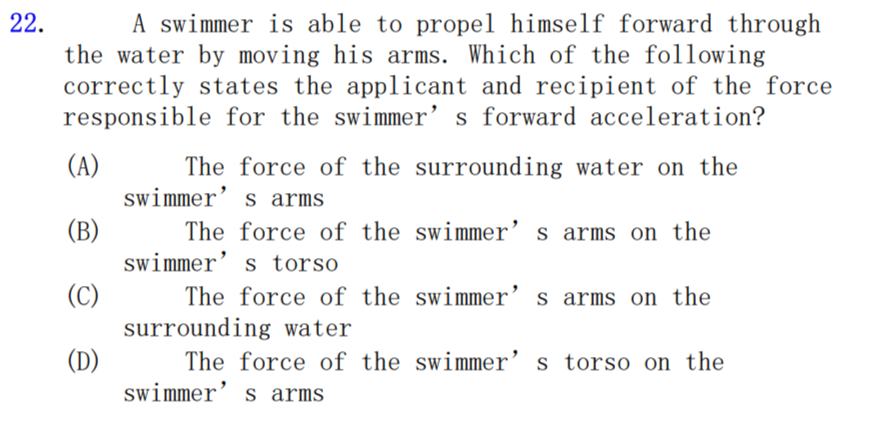A—Choice C is not correct because if the swimmer is accelerating, the responsible force must act on the swimmer.  But a force provided by himself on the himself won’t accelerate him. Choices B and D do not consider a force external to the swimmer. The answer is A: Newton’s third law force pair to the force of the swimmer’s arms on the water.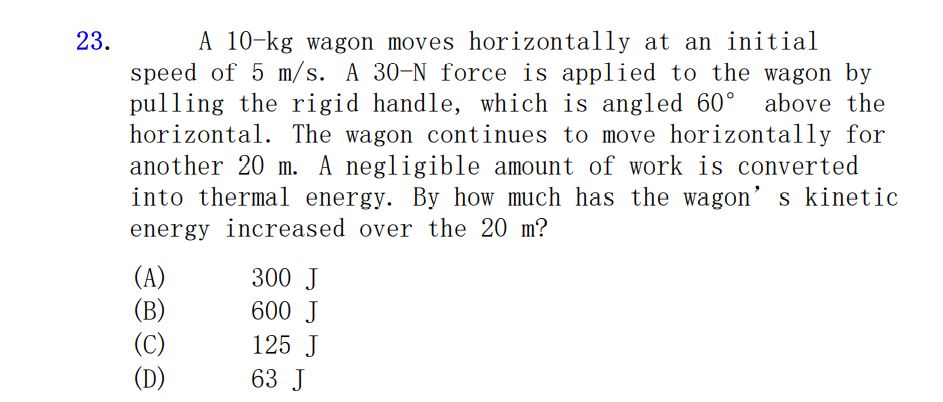A—The problem asks what is the same for each cart in comparison to the other. Since the carts are stuck together, they must move together. They have the same velocity as one another.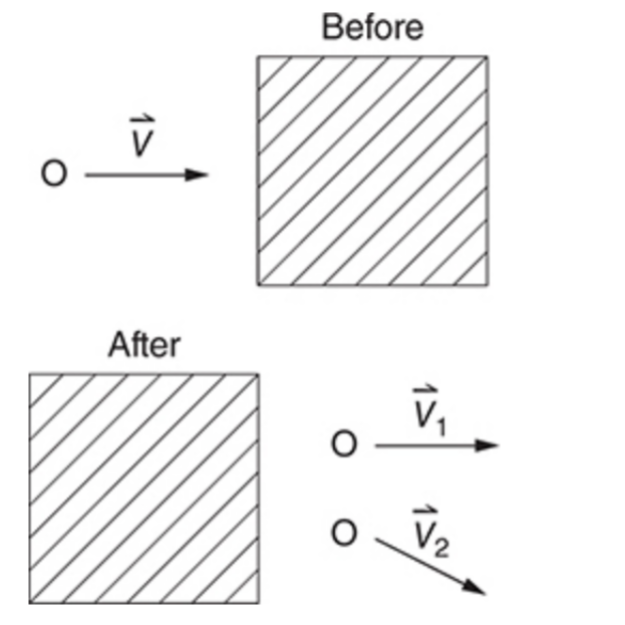### AP物理1选择题真题讲解第三期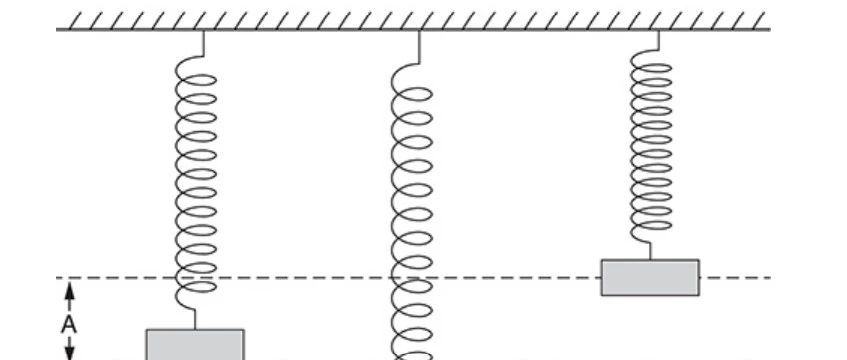5. ### IBDP课改后HL数学科目考什么？商务合作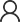在线咨询